# Compression ratio

Subjects:

• Compression ratio
• Calculating the compression ratio
• Limitations

Compression Ratio:
The compression ratio has a lot of influence on the performance of the engine. The higher it is, the higher the compression final pressure so the more energy the engine can extract from the fuel, thus producing more power. However, there are limitations to that. More on this later.

The compression ratio is a fixed ratio between the volume above the piston in ODP and the volume above the piston in TDC. The compression space is the volume of the combustion space in which the air is compressed by the piston. The compression space also depends on the thickness of the head gasket, the angle at which the valves are arranged in the cylinder head, the space occupied by the spark plug and injector. This makes it difficult to calculate. Therefore, the compression space is measured by pouring a certain amount of liquid into the head with the valves closed and measuring this amount.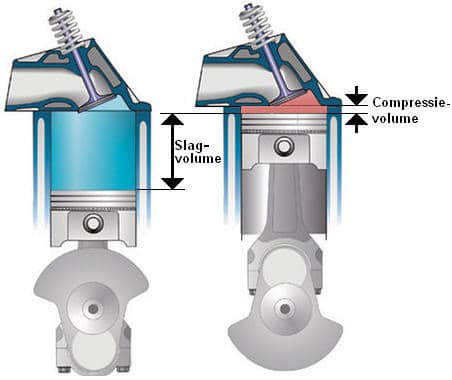Calculating the compression ratio:
The stroke volume is denoted by Vs and the compression volume by Vc. Both are in cubic centimeters (cm³). With these two data the compression ratio can be calculated. The compression ratio is indicated by the Greek letter ε (Epsilon).The engine data is as follows:
VS = 460 cm³
Vc = 50 cm³

Filled in this gives: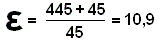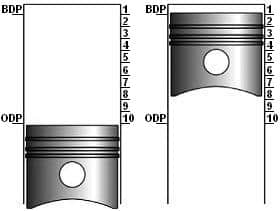Calculating this formula yields the answer 10. That means this engine has a compression ratio of 10:1. The figure below clearly shows the relationship between the volume above the piston in ODP (10) and the volume above the piston in TDC (1). The stroke volume + compression space are 10 times as large as the compression space.

An indirect injection petrol engine often has a compression ratio between 7:1 and 11:1. A direct injection petrol engine between 14:1 and 20:1.
The compression ratio of a diesel engine is often between 18:1 and 24:1.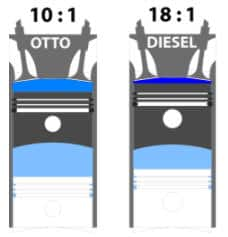As explained earlier, the volume of compression (Vc) is measured because it is very difficult to calculate. Stroke volume can be calculated. The formula is shown on the right.

π (pi) = rounded 3,14
d² = the diameter of the cylinder squared
s = the stroke in millimeters

In the standard compression ratio formula, Vs is replaced by the formula used to calculate Vs. The brackets to the left and right of the Vs formula indicate that this calculation must be done first. The result of this must be added to Vc and then divided by Vc.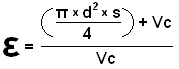As an example, let's take an engine with a bore x stroke of 81,0 x 86,4 mm. The bore is the diameter of the cylinder to be squared and the stroke is the distance the piston travels from ODP to TDC. The compression volume of this engine is given: 45 cm³.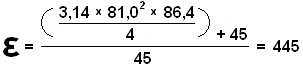Of course, the formula can also be implemented in steps. Entering this data into the formula gives: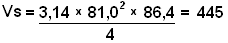The displacement of this engine can now be entered into the compression ratio formula:Limits:
With a higher compression ratio, more power can be achieved. This is because more energy can be extracted from the fuel (a higher efficiency). The compression ratio cannot simply be increased; there is a risk of knocking due to the higher compression final pressure. Due to the higher pressure and temperature, the fuel ignites earlier than intended. An engine equipped with a turbo has a higher compression end pressure due to the supercharging. With the same compression ratio, that would mean there is a risk of knocking. Therefore, the compression ratio in turbocharged engines is lower than in naturally aspirated engines.

Car manufacturers also use techniques to increase the compression ratio without the risk of knocking. Think of a knock sensor to advance the ignition (which is present on every engine these days), water injection to cool the combustion chamber, other fuels such as methanol and ethanol (this is used in racing).

Related page:error: Alert: Content is protected !!